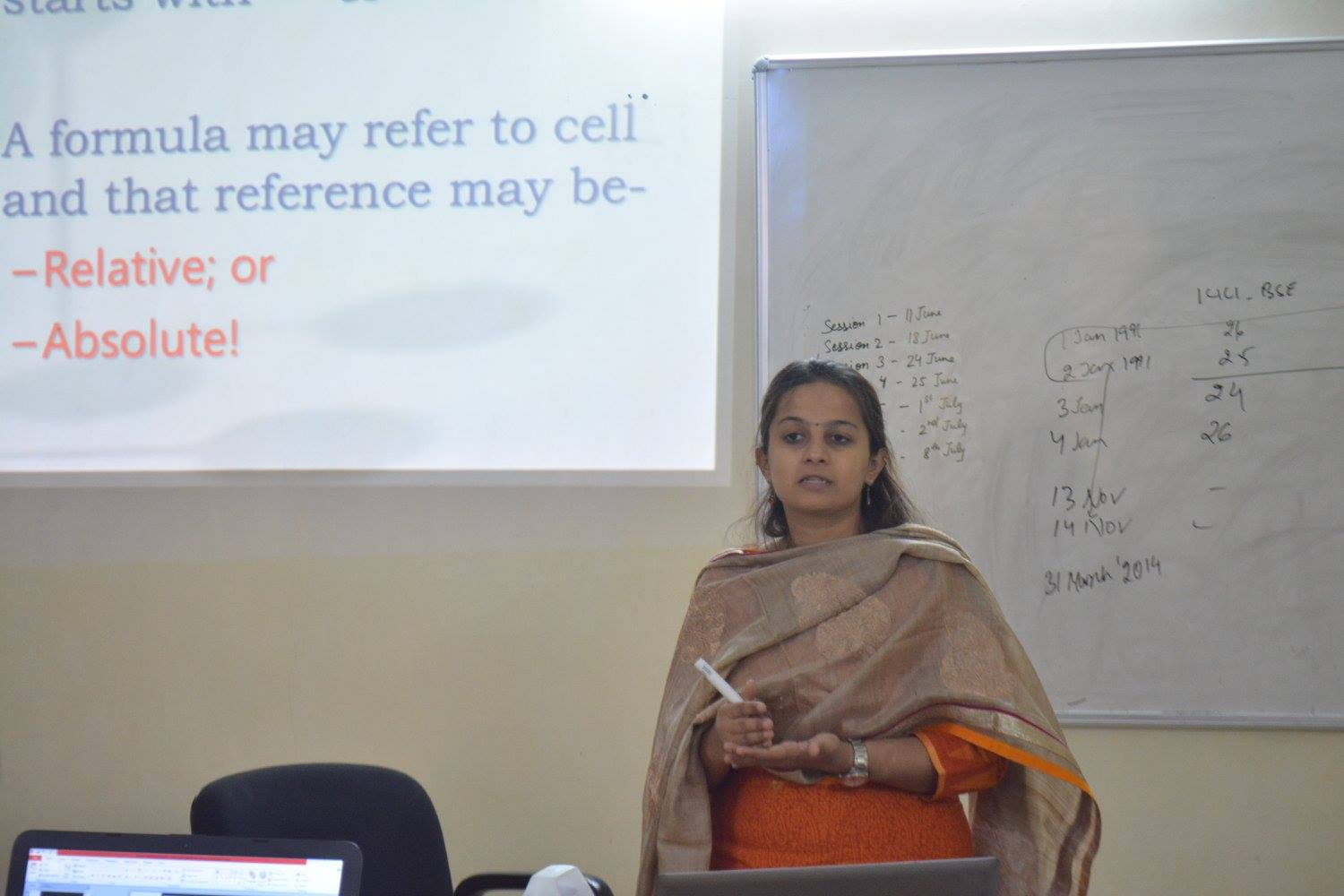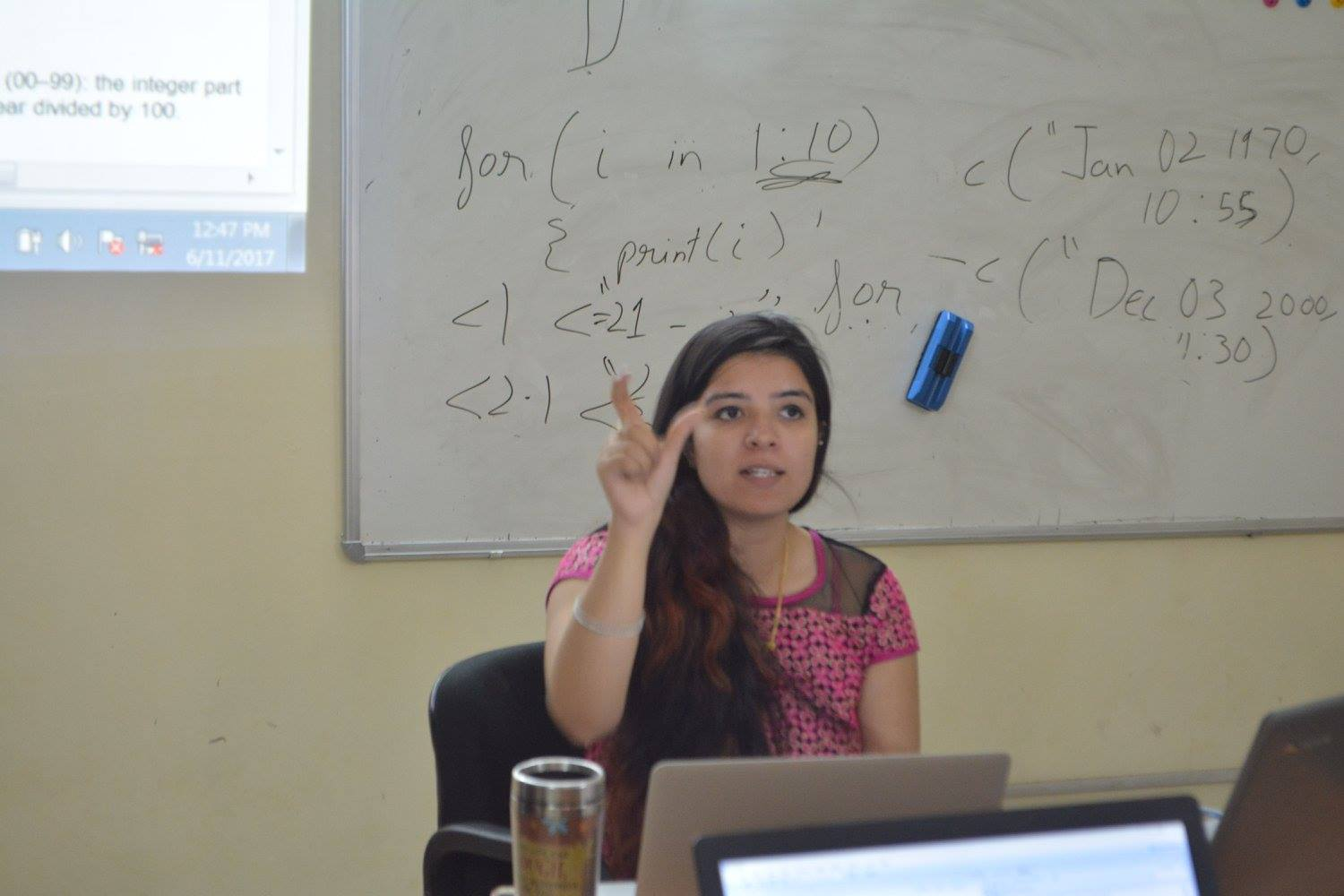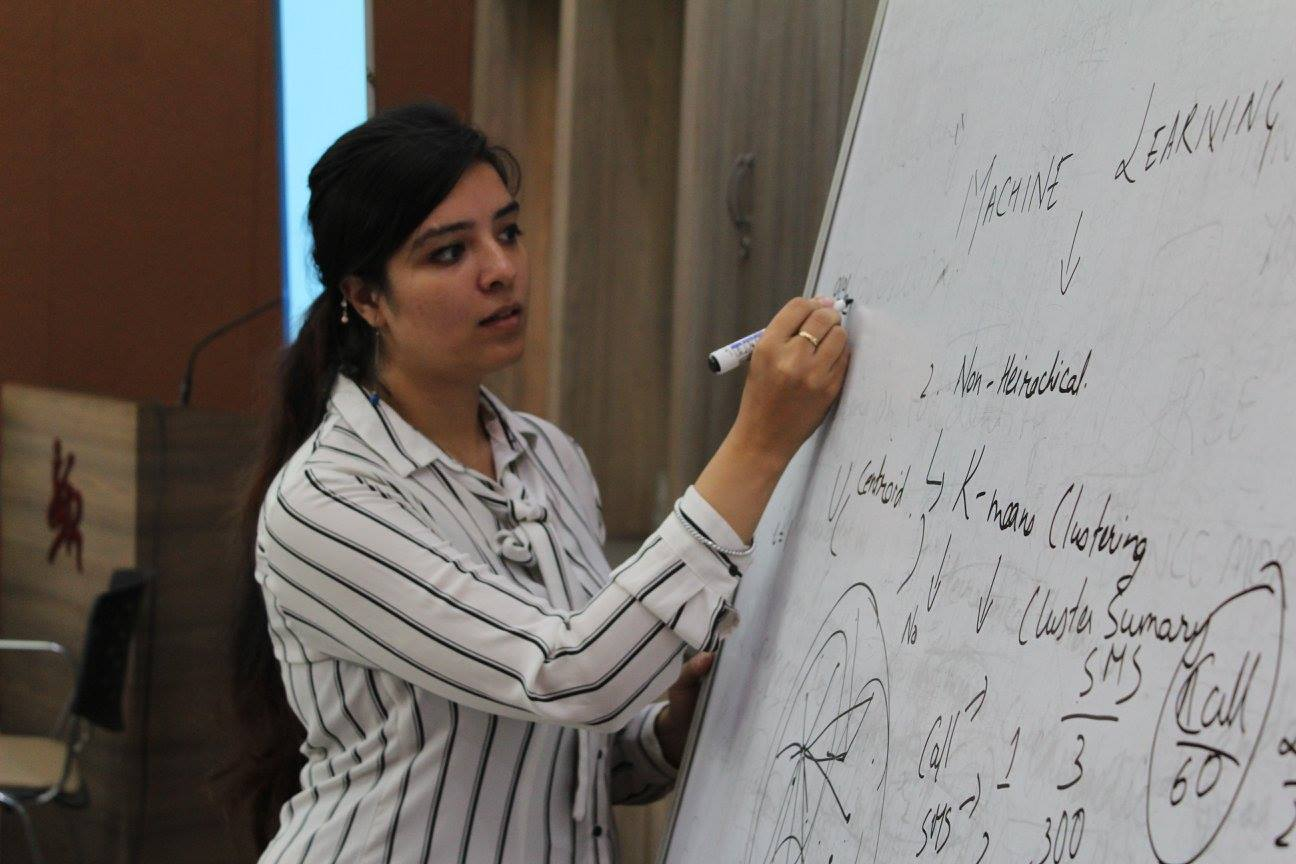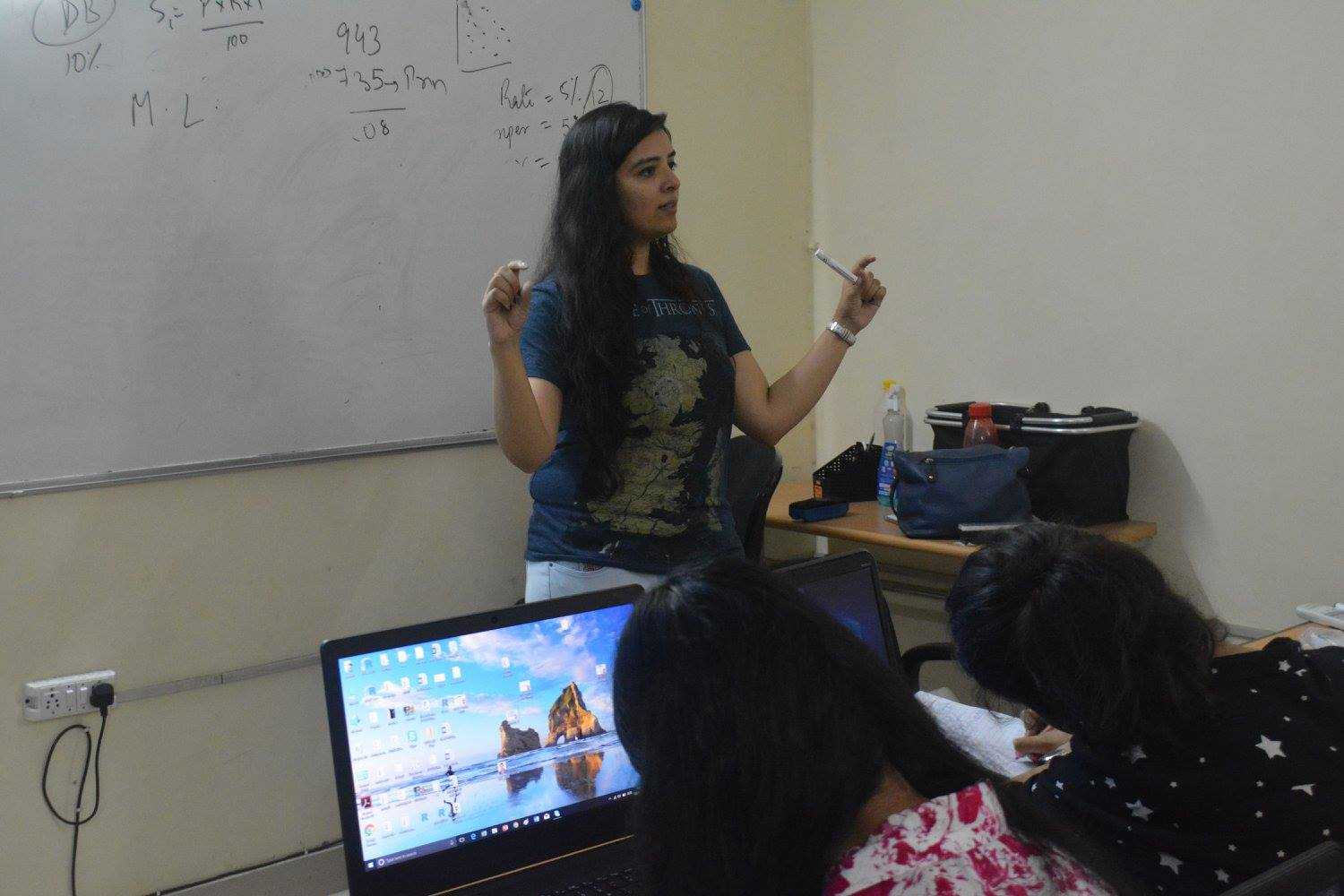## R

••••#### R

This course will introduce to R programming and it's implementation in data science. It also provides flavour of complete end to end journey of any data science project i.e. connection of R with data sources, manipulation and data engineering, exploratory analysis and visualisations, predictive modelling and connection to any other platform.

#### Prerequisites

Basic Knowledge of Statistics will be helpful

#### Faculty Profile

She is a statistician passionate about leveraging data science for mining out meaningful insights for business. she has experience of working for different business industries like BFSI, edutech, e-commerce, aviation, telecom and foodtech. Her Professional Skills include Probability, Statistics, Machine Learning, PostgreSQL, R, SPSS, SAS, Machine learning, Hive, Elastic search, Kibana and Python. She is presently working with Thevalley.nl and has worked in past with organizations like Zomato, Alqimi and Transorg in Data Scientist roles. She was also a Research Associate at IIIT Delhi.

#### Getting Started with R

• About the Software - History and Overview
• Installation
• Getting Familiar with R Environment

#### Programming in R : Part 1

R Nuts and Bolts

• Essentials
• Entering Input
• Evaluation
• R Objects
• Numbers
• Attributes
• Creating Vectors
• Mixing Objects
• Explicit Coercion
• Matrices
• Lists
• Factors
• Missing Values
• Data Frames
• Names
• Summary

Getting Data In and Out of R

• Using Textual and Binary formats for Storing Data
• Interfaces to Outside World
• Reading Lines of a Text File
• Reading Data from Internet and URL Connections

#### Programming in R : Part 2

Subsetting R Objects

• Subsetting a Vector
• Subsetting a Matrix
• Subsetting Lists

Vectorized Operations

Dates and Times

• Dates in R
• Times in R
• Operations on Dates and Times

Control Structures

• if-else
• for Loops
• Nested for Loops
• while Loops
• repeat Loops
• next, break

apply Family of Functions

• lapply
• sapply
• apply
• tapply
• split
• mapply

Sampling in R

• Simulation
• Random Sampling

#### Exploratory Data Analysis (EDA)

Basics of Distribution of Data

EDA for Individual Variables:

• Summarization: Measures of Central Tendancy, Dispersion, Skewness and Kurtosis
• Data Visualization: Histogram/Bar Chart, Box Plot, Stem and Leaf Display
• Missing Value Imputation
• Outlier Detection
• Testing for Normality: Histogram, QQ Plot, KS Test and SW Test

EDA for Multiple Variables:

• Pairwise Scatter Plots
• Correlation Analysis

Case Study: EDA for Motor Trend Car Road Tests Dataset

#### Statistical Inference

Parameter Estimation

• Parametric Estimation
• Non-Parametric Estimation

Parametric Testing of Hypothesis

• Testing for Hypothetical Value of Population Mean
• Testing for Equality of Two Population Means
• Testing for Hypothetical Value of Population Variance
• Testing for Equality of Two Population Variances
• Testing for Equality of Several Population Means

Non-Parametric Testing of Hypothesis

• Testing for Hypothetical Value of Population Median
• Testing for Equality of Two Populations
• Testing for Equality of Several Populations
• Testing for Goodness of Fit
• Testing for Independence of Attributes

Case Study: Parametric and Non-Parametric Tests

#### Linear Regression Analysis

Model Building

• Fitting a Linear Regression Model
• Testing the Significance of Individual Regressors and Overall Regression
• Goodness of the Model: R Square and Adjusted R Square

Multicolloinearity

• Problem and its Consequences
• Detection and Removal of Multicollinearity using Correlation Analysis
• Detection and Removal of Multicollinearity using Variance Inflation Factors (VIFs)

Parsimonious Modelling or Model Selection

• Forward Selection
• Backward Elimination
• Stepwise Selection

Validation of Assumptions and Residual Analysis

• Linearity of Regression
• Autocorrelation
• Heteroscedasticity
• Normality of Errors
• Outliers Detection

Case Study: Regression Analysis for Motor Trend Car Road Tests Dataset

#### Logistic Regression Analysis

• Fitting a Logistic Regression Model
• Testing the Significance of Individual Regressors and Overall Regression
• Goodness of the Model: Confusion Matrix, Sensitivity and Specificity
• Odds Ratio
• Multiclass Classification
• Case Study: Logistic Regression Analysis for Students’ Admission Dataset

#### Forecasting and Time Series Analysis

Estimating and eliminating the deterministic components if they are present in the model

• Testing for Presence of Trend - Relative Ordering Test
• Estimation and Elimination of Trend - Small Trend Method, Least Squares Method, Moving Averages Method
• Testing for Presence of Seasonality - Friedman (JASA) Test
• Estimation and Elimination of Seasonality - Small Trend Method, Large Trend Method

Modeling the residual using Auto Regressive Integrated Moving Average (ARIMA) model

• Testing for ‘stationarity’ using Augmented Dickey Fuller (ADF) Test
• Identifying the ‘order’ of the ARMA model using Correlogram, Partial Correlogram and Akaike Information Criterion (AIC)
• ‘Forecasting’ or predicting future values using Naive, Moving Average, Growth, Random Walk with Drift forecast.

Case Study - Forecasting and Time Series Analysis for Air Passengers Data